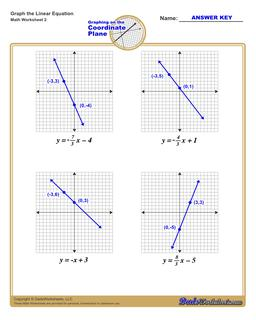One Dad. Four daughters. 9,341 worksheets... and counting!# Graphing Linear Equations (Second Worksheet)

PropertyValue
DescriptionGraphing Linear Equations: Each graphing linear equations worksheet on this page has four coordinate planes and equations in slope-intercept form, and includes an answer key showing the correct graph. (Second Worksheet)
Resource TypeWorksheet